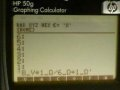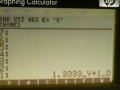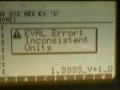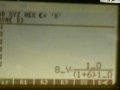#### Dafinc

Joined Feb 16, 2018
4

#### ericgibbs

Joined Jan 29, 2010
8,865
hi Dafinc,
Welcome to AAC.
Is this an Homework assignment, if Yes, I can move it to the Homework Forum.
E

#### Dafinc

Joined Feb 16, 2018
4
hi Dafinc,
Welcome to AAC.
Is this an Homework assignment, if Yes, I can move it to the Homework Forum.
E
Yes it is.
that would be awesome

#### AlbertHall

Joined Jun 4, 2014
8,513
Your formula is almost correct, it just needs two brackets to change the order of calculation.

#### LesJones

Joined Jan 8, 2017
2,349
Since when did 8 divided by 7 = 2.3333
Try doing the division again. First longhand on paper and then do it with a calculator.

Les

#### Dafinc

Joined Feb 16, 2018
4
Your formula is almost correct, it just needs two brackets to change the order of calculation.
so something like this ?

Vo = 8 * (1/6+1) =

#### AlbertHall

Joined Jun 4, 2014
8,513
Since when did 8 divided by 7 = 2.3333
Try doing the division again. First longhand on paper and then do it with a calculator.

Les
but 8 / 6 + 1 does equal 2.3333

#### LesJones

Joined Jan 8, 2017
2,349
Hi Albert,
Yes, I agree it is ambigious without the brackets.

Les.

#### Dafinc

Joined Feb 16, 2018
4
I think i got the right answer now.

instead of typing 8 * 1/6+1 = 2,33

i typed 8 * 1/7 which gave me the value 1,1429V

which is the same value as the calculator gives me.

#### InPhase277

Joined Feb 15, 2018
23
The order of mathematical operations is fundamentally an artificial construct, but we have to agree across the board, so someone in the past set the standards: PEMDAS

Parentheses first, Exponents, Multiplication as they appear, Divisions as they appear, Additions and Subtraction as they appear.

#### Bordodynov

Joined May 20, 2015
2,419
I think the problem is inattention.
Stop mocking the man!
What I show below is also a learning process. If the author of the topic understands that I wrote, it will be progress in learning.
Vo = 8V * 1ohm/(6ohm + 1ohm) = 8V/1Ohm/7Ohm=1,142857142857V
i=Vs/Rser, Rser=Rp+Rs, Vo=i*Rp=Vs/Rser*Rp=Vs*Rp/Rser=Vs*Rp/(Rp+Rs)

#### dl324

Joined Mar 30, 2015
9,122
instead of typing 8 * 1/6+1 = 2,33

i typed 8 * 1/7 which gave me the value 1,1429V
Either you need to learn how to do arithmetic or you need to learn how to use a calculator.

The equation you're trying to solve is:
$$\small Vo = Vs * \frac{Rp}{Rp+Rs} Vo = 8V * \frac{1 \Omega}{1 \Omega + 6 \Omega} = 8V * \frac{1}{7} = 1.14V$$

If you had kept track of units, you would have noticed that the equation first used wouldn't give Volts. This would: 8V * 1Ω/(6Ω + 1Ω).

#### InPhase277

Joined Feb 15, 2018
23
If you had kept track of units, you would have noticed that the equation first used wouldn't give Volts. This would: 8V * 1Ω/(6Ω + 1Ω).
One of the biggest problems that I see made with math involving physical units is that people forget that the units themselves have to undergo the mathematical massaging just like the numbers.

One of the first things to do with an incorrect answer is check to see if it is dimensionally correct.

#### shteii01

Joined Feb 19, 2010
4,647
8*1/(6+1)=1.14
_____^ normal people do it this way

#### MrAl

Joined Jun 17, 2014
6,615
i have to apply voltage division to this circuit to express the Output voltage across the Rp resistor.
View attachment 146135
these are my calculations but i cant figure out if they are true or if it is the right way to do it:

Rs = 6 Ohm.
Rp = 1 Ohm
Vs = 8V

Vo = 8V * 1ohm/6ohm + 1ohm = 2,3333 V

Using the Calculator on this website gives me a different value.
Hi,

Just a little note here...

Normally when we do a voltage divider we use the formula:
Vout=Vin*Rd/(Rd+Ru)

where when the circuit is drawn like that:
Rd is the lower resistor (down),
Ru is the upper resistor

This gets you there quick.
(I think you started to do this right but just didnt do the math right perhaps.)

You might ask the question why this works though.
The reason is because when the output voltage is solved as above, the current through BOTH resistors is the same.
You can look at Kirchoff's Laws to find out more.

#### WBahn

Joined Mar 31, 2012
24,853
i have to apply voltage division to this circuit to express the Output voltage across the Rp resistor.
View attachment 146135
these are my calculations but i cant figure out if they are true or if it is the right way to do it:

Rs = 6 Ohm.
Rp = 1 Ohm
Vs = 8V

Vo = 8V * 1ohm/6ohm + 1ohm = 2,3333 V

Using the Calculator on this website gives me a different value.
In order to get this result, you are interpreting the equation as

Vo = (8 V * 1 Ω / 6 Ω) + 1 Ω

However, while the expression in parentheses is 1.667 V, you then need to add this to 1 Ω and you can't add a voltage to a resistance. It's like asking what 3 feet added to 2 pounds is. It's fundamentally nonsensical.

So the biggest, most important lesson you can possibly learn from this exercise is to always, always, ALWAYS properly track your units. Most mistakes you make will mess up the units, as your mistake here did, but only if you track them. You can't just tack on the units that you want the answer to have at the end, which is what you did here.

This is actually a very, very common mistake. Humans are used to writing

$$A \; = \; \frac{B}{C+D}$$

and when we then put this in an in-line text form there is a natural tendency to just replace the horizontal line with a forward slash and get

A = B/C+D

But the horizontal line did more than just indicate a division operation, it also acted as a grouping operator, thus the proper translation should be

A = (B) / (C+D)

Without those parens, we have

A = (B/C) + D

which is a very, very different expression.

Now, sadly, no calculator that I am aware of lets you track units. There have been some computer programs that have at least tried to do that, but I've never run across them. So when you go to plug numbers into the calculator, you have to understand it's order of operations well enough to know that it is doing what you want and, if not, how to override them to force it to do what you want. One of the best things you can do is estimate what you expect the answer to be before you plug the numbers into the calculator and if the value you get when you do isn't in line with it -- stop. Figure out whether you are using the calculator incorrectly or you are estimating poorly. In this case you have an 8 V source supplying current to a circuit with a total of 7 Ω, so the current is just a little more than 1 A. The voltage across the 1 Ω output resistor is thus going to be just a little more than 1 V. It is NOT going to be something over 2 V, so when you get that answer, all kinds of red flags should go up.

So:

#### WBahn

Joined Mar 31, 2012
24,853
The order of mathematical operations is fundamentally an artificial construct, but we have to agree across the board, so someone in the past set the standards: PEMDAS

Parentheses first, Exponents, Multiplication as they appear, Divisions as they appear, Additions and Subtraction as they appear.

So, according to your description, the following expression

X = 64 / 4 * 8

should yield a value of 2.

There are no parens or exponents.

You then say Multiplication as they appear. That means it becomes

X = 64 / (4 * 8)

But the universally (as far as I am aware) agreed upon rules for order of operations state that multiplication and division are of the same precedence and are left associative and that, likewise, addition and subtraction are of the same, albeit lower, precedence and also left associative.

Thus

X = 64 / 4 * 8 = (64 / 4) * 8 = 128

#### WBahn

Joined Mar 31, 2012
24,853
I think the problem is inattention.
Stop mocking the man!
What I show below is also a learning process. If the author of the topic understands that I wrote, it will be progress in learning.
Vo = 8V * 1ohm/(6ohm + 1ohm) = 8V/1Ohm/7Ohm=1,142857142857V
i=Vs/Rser, Rser=Rp+Rs, Vo=i*Rp=Vs/Rser*Rp=Vs*Rp/Rser=Vs*Rp/(Rp+Rs)
You might want to review your equations. You have a typo there.

#### The Electrician

Joined Oct 9, 2007
2,750
Now, sadly, no calculator that I am aware of lets you track units.
There was a TV comedian who would have said to you: "You don't get out enough!"

The 25+ year old HP48 calculator could track units and a number of its descendants still do. Here's the TS's calculation as he typed it in, shown on my HP50G calculator. Sorry for the slightly blurry images:Now if I press the "ENTER" key the calculator does some calculations, keeping units:Now I press the "EVAL" key in an attempt to combine units:But if I type in this:And then press "EVAL", I get this:#### JoeJester

Joined Apr 26, 2005
4,126
I see the third image as something @WBahn could use a lot in the homework help area ....